﻿ NVector2DF Structure Members
Nevron .NET Vision
Nevron.GraphicsCore Namespace / NVector2DF Structure
Fields Properties Methods

In This Topic
NVector2DF Structure Members
In This Topic

The following tables list the members exposed by NVector2DF.

Public Constructors
NameDescription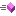Overloaded.
Top
Public Fields
NameDescription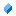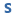The empty (zero) vectorThe X unit vectorThe Y unit vectorX coordinateY coordinate
Top
Public Properties
NameDescription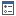Determines whether the vector is the unit vector (e.g. Length == 1)Gets the vector lenghtGets the vector lenght square (length * length)Gets the unit vector (with length == 1), which is perpendicular to this vectorGets a vector, which is perpendicular to this vector
Top
Public Methods
NameDescriptionPerforms a scalar division of a vectorComputes the dot product of this vector with another vectorServes as a hash function for a particular type, suitable for use in hashing algorithms and data structures like a hash table.Inverses this vector (e.g. makes this vector with oposite direction)Performs a scalar multiplication of this vector with the specified scalar and returns the resulting vectorMakes this vector the unit vector (e.g. its length becomes 1)Computes the perpendicular dot product of this vector with another vectorConverts this vector to NPointFConverts this vector to PointFReturns a System.String that represents the current System.Object.
Top
Public Operators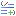Addition Adds b coordinates to a coordinates and returns the resulting vector (binary +)Equality Determines whether a is equal to bInequality Determines whether a is not equal to bSubtraction Subtracts b coordinates from a coordinates and returns the resulting vector (binary -)
Top